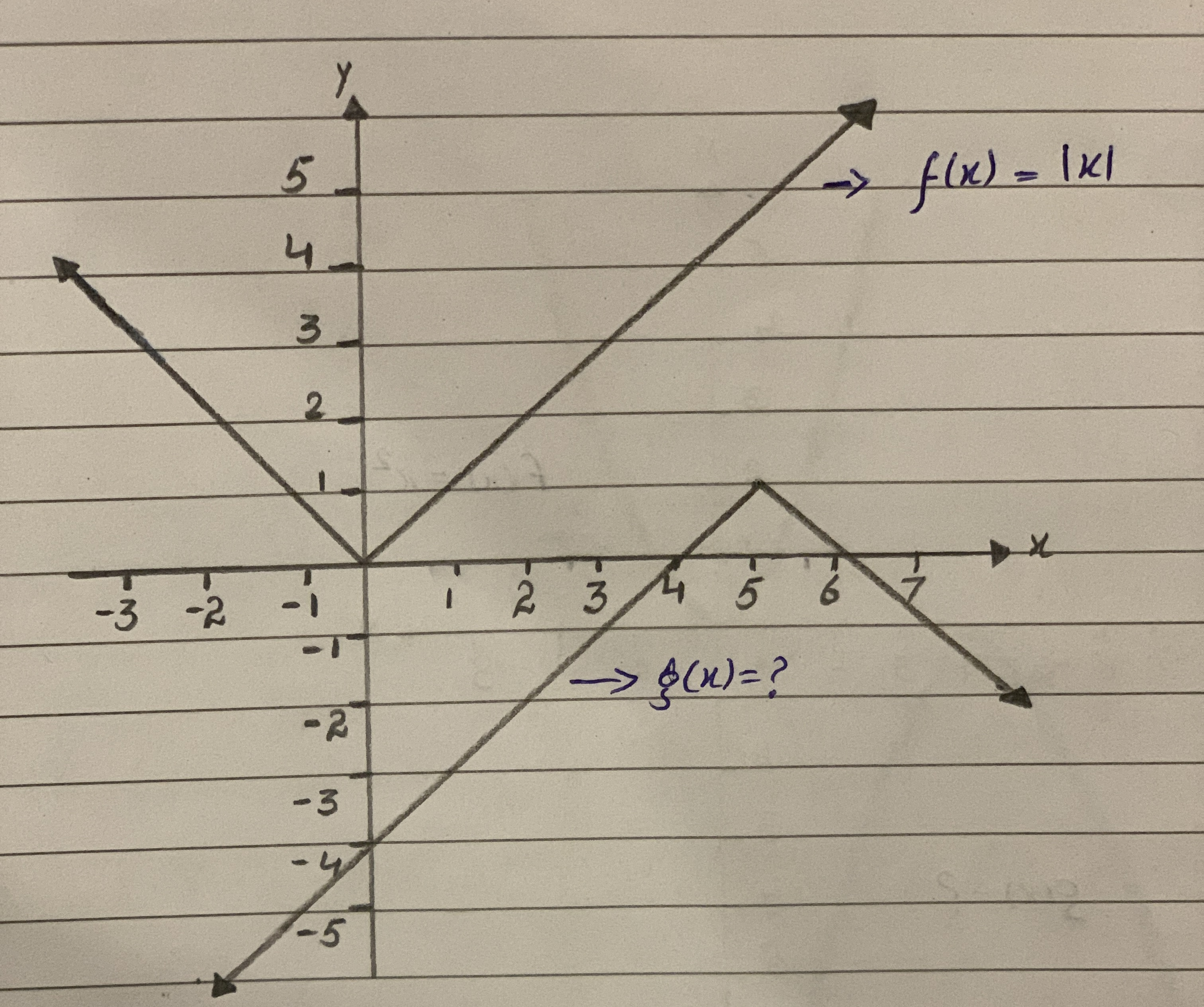Functions f and g are graphed in the same rectangular coordinate system. If g is obtained from f through a sequence of transformations, find an equation for g. (Graph given on the link)illusiia 2020-11-22 Answered
Functions f and g are graphed in the same rectangular coordinate system. If g is obtained from f through a sequence of transformations, find an equation for g.

• Questions are typically answered in as fast as 30 minutes

Solve your problem for the price of one coffee

• Math expert for every subject
• Pay only if we can solve itlobeflepnoumni

Given:
The graph of $$f(x) = sqrt(16−x^2)$$
To determine :
The equation of transformed graph shown in the given figure.
The parent graph of $$f(x) = sqrt(16−x^2)$$.
Since the graph is shifted vertically down by 1 unit.
Therefore, the equation becomes,
$$f(x) =sqrt(16-x^2)-1$$
Also, the graph is a reflected graph in the x - axis . Hence we have
$$f(x) =sqrt(16−x^2)− 1$$
Now, the graph is has a vertical compression by 4 units.
Therefore, the transformed graph is $$f(x) =− 1/4sqrt(16-x^2)-1$$